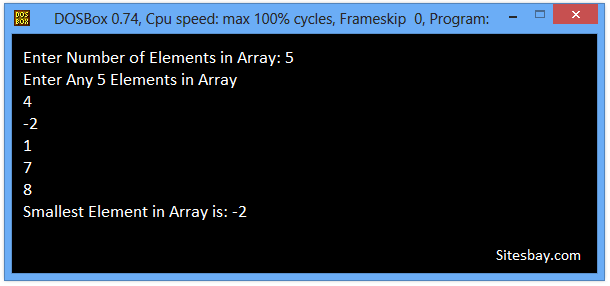# C Program to Find Smallest Element in Array

## Find Smallest Element in Array Program in C

To write this code we receive input from user and store in array variable after that sort array elements and find smalles element.In this Code, we will find the smallest element of array by using linear search. Given an array of N elements, we have to find the Smallest Element of Array.

## Example

```Array : [29, 12, 11, -16, -14, 13, 0, -3]
Smallest Element : -6
```

To understand below example, you have must knowledge of following C programming topics; String in C, for looping statements we need know For Loop in C and Array in C.

### Algorithm to Find Smallest Element of Array

• First take input from user as a size of Array
• Then ask user to enter elements in Array
• Initialize one variables smallest with first element of inputArray.
• Using Loop traverse array from 0 to N-1 and compare each element with smallest. If current element is less than smallest, then update smallest with current element.
• After array traversal smallest will have the smallest element.

## C Program to Find Smallest Elements from Array

```#include<stdio.h>
#include<conio.h>

void main()
{
int array, count, i, smallest;
clrscr();
printf("Enter Number of Elements Want in Array\n");
scanf("%d",&count);

printf("Enter Any %d count Elemnts in Array \n", count);

for(i = 0; i < count; i++){
scanf("%d",&array[i]);
}

smallest = array;
// search num in inputArray from index 0 to elementCount-1
for(i = 0; i < count; i++){
if(array[i] < smallest){
smallest = array[i];
}
}

printf("Smallest Element in Array is: %d",smallest);

getch();
}
```

## Output

```Enter Number of Elements in Array: 5
Enter Any 5 Elements in Array
4
-2
1
7
8
Smallest Element in Array is: -2
```WIFI CCTV Camera